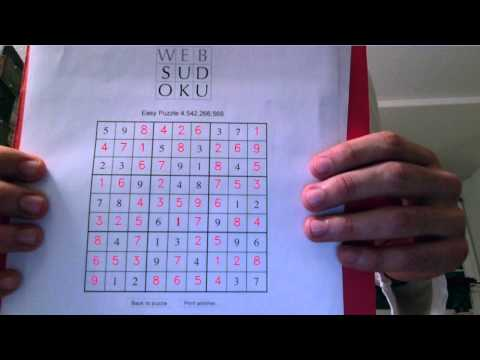### Jordi Pont-Tuset

I'm from a small town near Girona, Catalunya. Currently in Zürich, Switzerland. Passionate about computer vision, technology, running, and mountains.

# Solving Sudoku puzzles like a pro (part I)

In the following tutorials I’ll explain how to solve Sudoku puzzles using C++ and OpenCV. We’ll build a program that captures the webcam video, looks for a Sudoku grid, locates and recognizes the numbers, solves the puzzle, and reprojects the result on the grid (augmented-reality); all in real time. Spoiler alert! Here the link to the final result video :)This first part will be about locating the grid and numbers. You can download the full code from GitHub, here I’ll just highlight the main parts to understand the algorithm. Please note that the proposed algorithm is not the optimal in a lot of senses, since it’s been designed as an academic example that one can understand without a lot of knowledge about computer vision. This has left out some powerful but more advanced techniques.

#### 1. Capture and show the webcam feed

First, let’s capture and show the camera feed, the code is pretty self-explanatory.We’ve got our image from the webcam, let’s get ours hands on it.

#### 2. Detect contours using Canny’s edge detector and lines using the Hough transform

We know that a Sudoku grid is characterized by a certain pattern of lines, and a line is a straight segment of contours, so the first step we want is to detect the contours in the image. There is a whole world in the literature about how to detect contours, but we’ll use the simplest and most known: the Canny edge detector. Once we have the contours, we’ll look for sets of contours forming a line, using the Hough line transform.The result of the contour detector is a binary image where each pixel is classified either as being contour (white) or not (black). Now we would like to find the lines in the image, that is, sets of white pixels that lay in a common line.

To do so, we’ll use the Hough transform. The intuitive idea behind this transform is that each contour pixel (white) vote for all lines that can pass through that point. We then gather all votes and those lines with more votes are considered as detected. The Hough transform is one of those must-know simple and neat ideas in Computer Vision, so if you’re not familiar with it, I suggest to take a look at how it works.#### 3. Filter lines and recognize the Sudoku grid

The result of the previous step is a set of lines detected in the image, represented by their slope and distance to the origin (\rho-\theta representation). The main advantage of this representation is that it can represent any line (no problems with vertical lines as in the y = ax+b representation), but as a drawback, the analytical treatment tends to be more complex.

We first classify the lines into horizontal, vertical, and others, setting thresholds to their slope. We will then sort them in order of their distance to the origin, which will help in next steps.To detect the Sudoku grid, we will look for a pattern of ten evenly distributed horizontal and vertical sets of lines. Instead of trying to look for the pattern among lines, we will work with the intersection between them, to make the algorithm more robust to noise in the line detection and the code easier to understand.

We therefore compute the pairwise intersection between all vertical and horizontal lines, and then we select a line in the center (as sorted before) and scan the intersection with the orthogonal lines. We then look for ten sets of evenly distributed points, and if we find them we classify the lines creating those points into the ten sets of Sudoku lines. If we find a correct horizontal and vertical sets of ten lines, we have found the Sudoku grid.#### 4. Extract all 81 Sudoku cells locations

Once we have recognized and located the 100 intersections between 10 horizontal and 10 vertical lines, we only need to extract the rectangles between all four neighboring intersections. We reduce the rectangle a little to be robust to noise and misalignments, and we have the 81 cells we were looking for.You can find the code from this blog post in GitHub. The rest of the algorithm is described in a second blog post here.